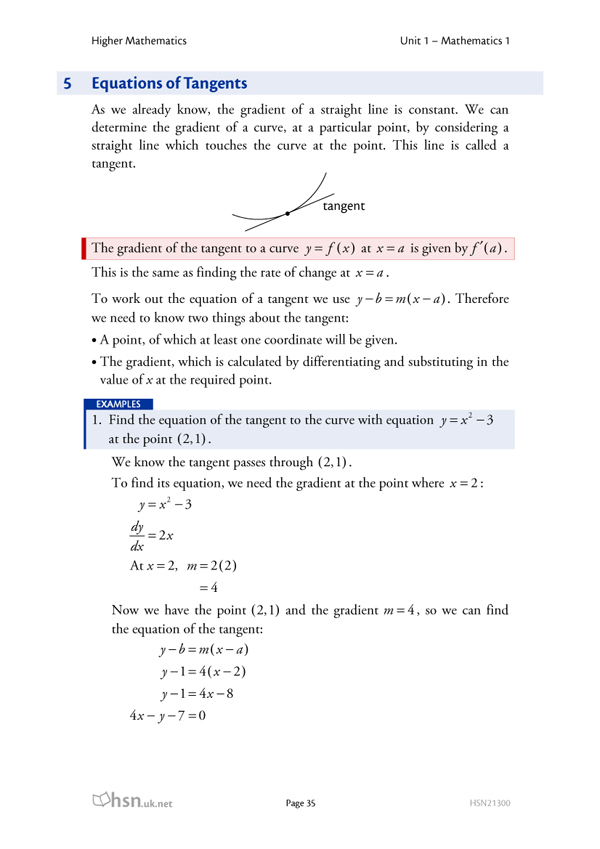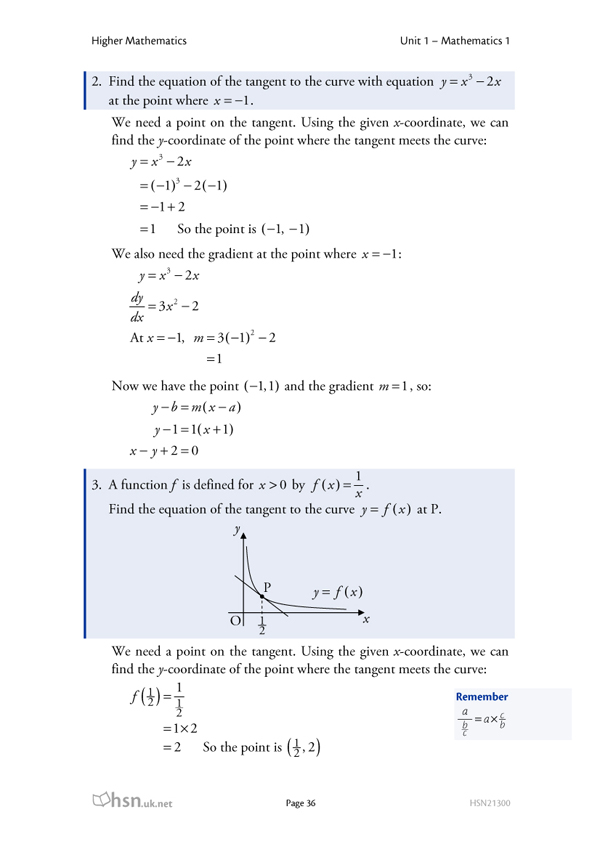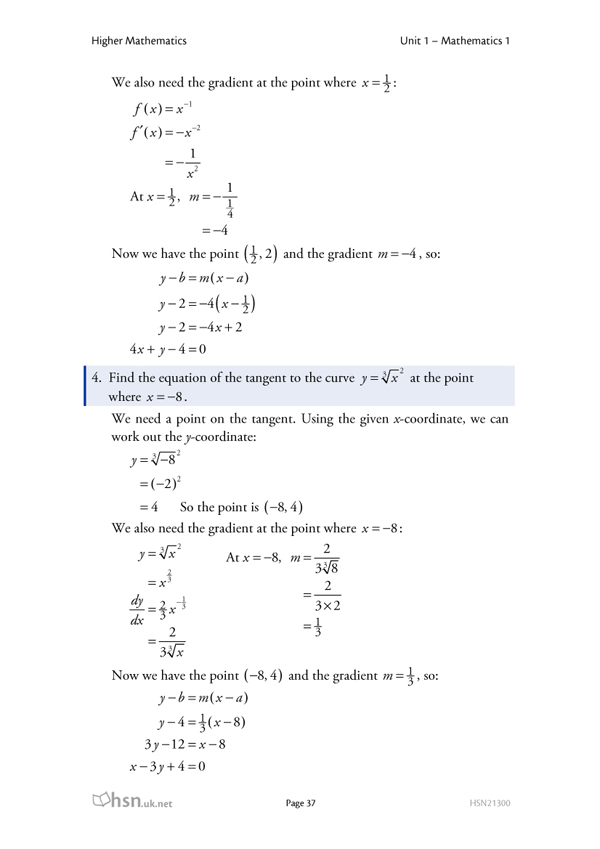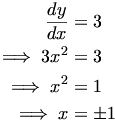Warning: Illegal string offset 'html' in /home/hsn/public_html/forum/cache/skin_cache/cacheid_1/skin_topic.php on line 909

Just a few questions on Curves and tangents.... - HSN forum# Just a few questions on Curves and tangents....

8 replies to this topic

### #1lynnie

• Gender:Female

Posted 25 September 2006 - 07:25 PM

1. A curve has equation y = x^3 - 4x^2 +2x -1
Find the equation of the tangent to the curve where x = 2

2. The point P(-1,7) lies on the curve with equation y = 5x^2 + 2
Find the equation of the tangent to the curve at P

I am totally lost with these and if i could jsut get some help it would really do me some good in completing the rest of this homework....due in tomorrow :S

Thanks a lot. X

### #2George

Child Prodigy

••• 720 posts
• Location:West Lothian
• Gender:Male

Posted 25 September 2006 - 07:39 PM

OK, don't panic - these questions aren't impossibleIn fact, there are some really similar examples in our free notes on differentiation - have a look at Section 5 - examples 1 and 2 are almost identical to these questions.

In each case, you're finding the equation of a tangent, and you need to be able to differentiate to do that.

If you're still stuck, just post again to say what it is you don't get.

### #3lynnie

• Gender:Female

Posted 25 September 2006 - 07:52 PM

Hi thanks...but the notes do not work on my comp for sum reason...:-s

Oh my god...I can't do any of this...

I need help. thanks xx

### #4George

Child Prodigy

••• 720 posts
• Location:West Lothian
• Gender:Male

Posted 25 September 2006 - 08:06 PM

OK, here are the relevant pages from the notes:### #5lynnie

• Gender:Female

Posted 25 September 2006 - 08:43 PM

Wow!! Thank you so much for posting they notes up! thank you thank you thank you etc..

Much appreciated.....but im bound to get stuck at the next one lol

x

...actually.....im really sorry about this by the way.....but Is there any notes on a question where you have the curve and this time just the gradient.....im soo sorry.....x or even if someone could jsut explain it. thanks

Top of the Class

•• Members
•• 390 posts
• Location:Cambridge
• Interests:Muzak.
• Gender:Male

Posted 25 September 2006 - 09:05 PM

I had a look through the notes but I couldn't find anything that seemed to fit what you are talking about (if you want to look at the notes yourself you need to download adobe acrobat reader http://www.adobe.com/products/acrobat/readstep2.html - this might be why you can't access the notes). It's definately worth making the effort because the notes because they are very useful.

Anyway, I'm not sure what you are asking here. I think you're saying that you are given the equation of the curve and the gradient of the tangent, and you have to find the equation of the line? I'll post how I would do this - if this isn't what you're looking for you may want to ignore this.

So you have the equation of your curve. If you differentiate this, you get a formula for the gradient at any point in the curve. So in your derivative (the derivative is what you get when you differentiate) you can put in a value of x, say x=1 and this will give you the gradient of the curve at that exact point. Now you know that a tangent to the curve has the same gradient as the curve at this point.

But what happens if we don't have an x value, but we have our derivative and the gradient of the tangent. Well all we have to is set the derivative equal to the gradient (after all, we are trying to find the point at which the curve has the same gradient as the line we're trying to find, so we set them equal). You should have an equation now that is solveable for x. The x value you find from here is the the point on the curve where the gradient of the curve is the same as the gradient of the tangent.

Now you have an x-value on your curve where the gradient is the same as the line you are trying to find. The y-value will also be the same, so put your x-value you just found into the original equation and solve for y. Now you will have a coordinate which is where your curve meets the line, so the point also lies on the line. You have a gradient and a coordinate, can you find the equation of the straight line from here?
HMFC - Founded 1874, beefing the Cabbage since 1875

### #7George

Child Prodigy

••• 720 posts
• Location:West Lothian
• Gender:Male

Posted 25 September 2006 - 09:15 PM

Glad that helpedI'm not sure what you mean by "you have the curve and this time just the gradient" - is it something like "Find the points on the curve y = x3 where the tangent has a gradient of 3"?

Here's how to tackle a question like that, just in case:gives you the gradient of the tangent. We need to find values of x so that.

Differentiating, we get, soThe last step is to find the coordinates of these points. The question could even ask for the equations of these lines, but that should be OK too since you have the points and the gradient.

If that's not what you were asking about, I hope I've not confused youEdit: Just noticed the previous post - obivously I recommend downloading the notes tooWhat ad absurdum said is basically describing the method I've used above.

### #8lynnie

• Gender:Female

Posted 25 September 2006 - 09:20 PM

The question was...

Find the equations of the tangents to the curve y = x^3 -9x + 4 which have gradient 3.

If that the explanation on how to do that, above? ^ ^

Sorry I should have posted the question to begin with.

Top of the Class

•• Members
•• 390 posts
• Location:Cambridge
• Interests:Muzak.
• Gender:Male

Posted 25 September 2006 - 09:20 PM

Edit: Just noticed the previous post - obivously I recommend downloading the notes tooWhat ad absurdum said is basically describing the method I've used above.
Great minds think alikeYeah lynnie that's the one, give it a goHMFC - Founded 1874, beefing the Cabbage since 1875

#### 1 user(s) are reading this topic

0 members, 1 guests, 0 anonymous users